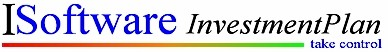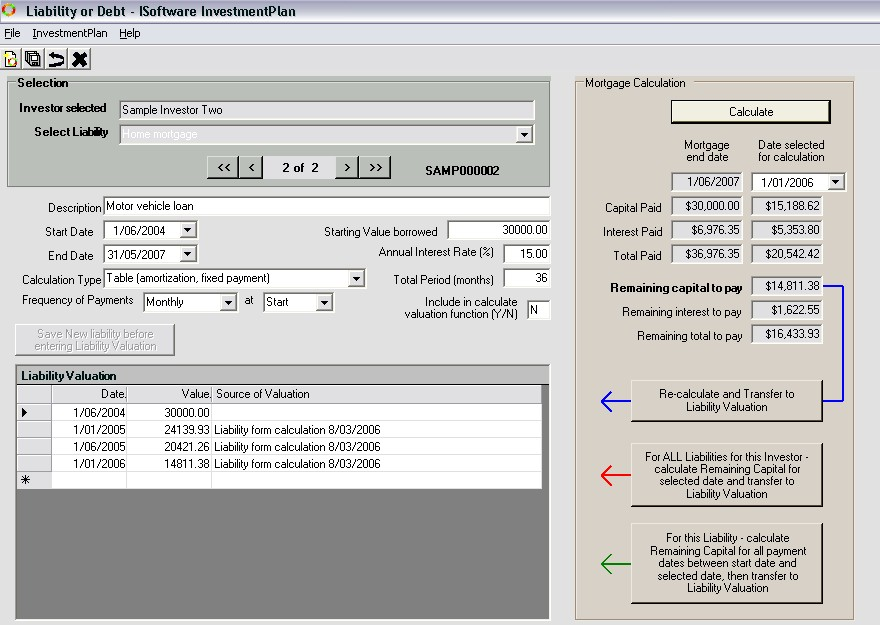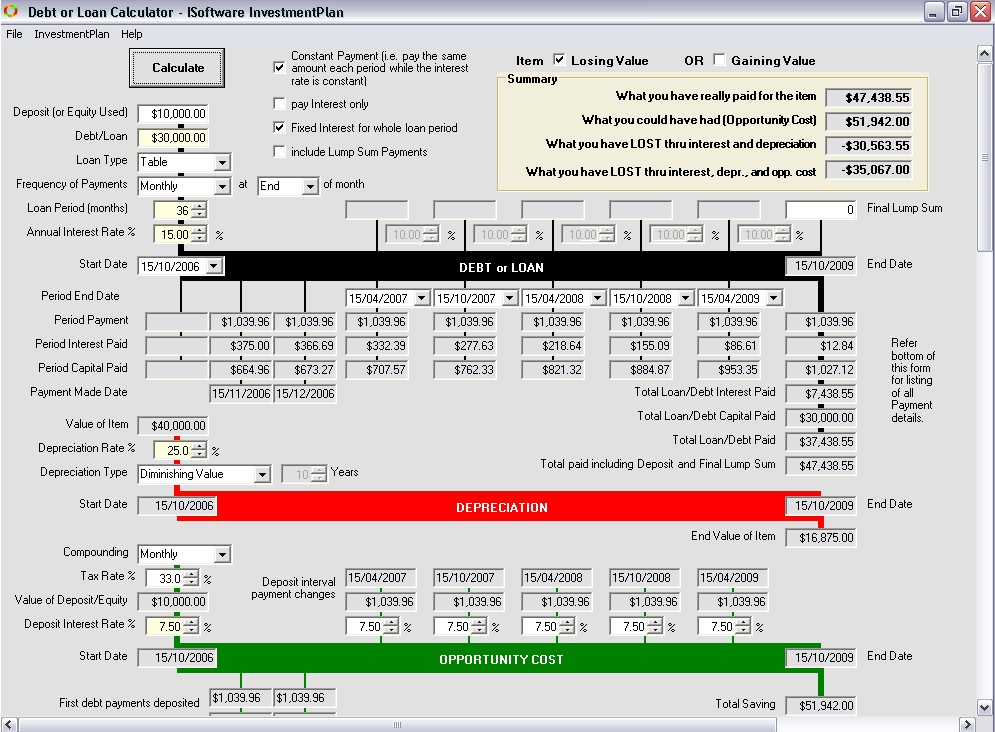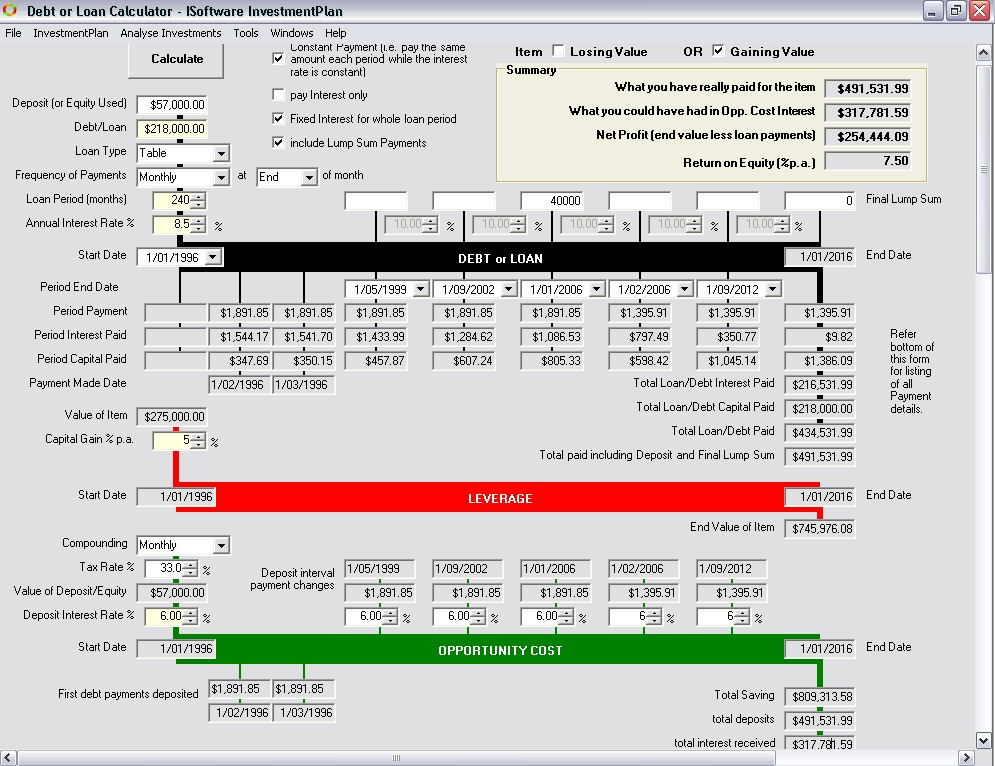#### Debt and mortgage calculations

Debt payments calculation, calculating the true cost of using debt either as leverage on growing assets, or total loss on depreciating items.

InvestmentPlan has a number of extremely flexible loan calculators.

• Allows for changing interest rates
• Can include lump sum payments.
• Allows a range of loan types, payment frequency, and when payments are made.
• Depreciation calculation of the item purchased, if the item is losing value (refer below)
• Leverage calculation of the item purchased, if the item is gaining value (refer below)
• Calculation of opportunity cost, including tax adjustments, and changing interest rates
Liability entryThe True cost of borrowing for depreciating items calculator (items losing value)

Example: Calculate the real cost of purchasing a \$40,000 car with a \$30,000 loanCalculate the benefits of borrowing for items increasing in value (leverage)

Example: Calculate the return and profit for a property which is an asset gaining value.